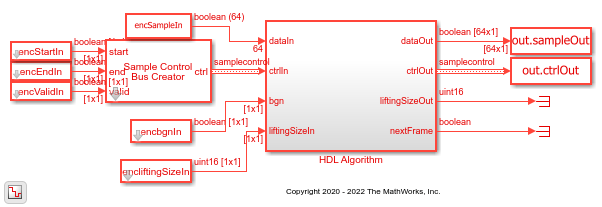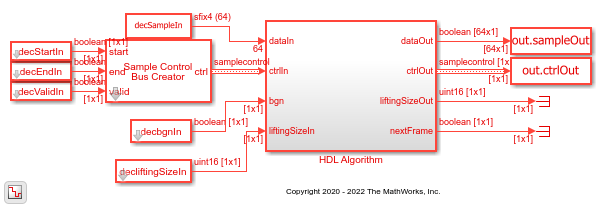# LDPC Encode and Decode of 5G NR Streaming Data

This example shows how to simulate the NR LDPC Encoder and NR LDPC Decoder Simulink® blocks and compare the hardware-optimized results with the results from the 5G Toolbox™ functions. These blocks support scalar and vector inputs. The NR LDPC Decoder block enables you to select either Min-sum or Normalized min-sum algorithm for decoding operation.

### Generate Input Data for Encoder

Choose a series of input values for bgn and liftingSize according to the 5G new radio (NR) standard. Generate the corresponding input vectors for the selected base graph number (bgn) and liftingSize values. Generate random frames of input data and convert them to Boolean data and control signal that indicates the frame boundaries. `encFrameGap` accommodates the latency of the NR LDPC Encoder block for bgn and liftingSize values. Use the nextFrame signal to determine when the block is ready to accept the start of the next input frame.

```bgn = [0; 1; 1; 0]; liftingSize = [4; 384; 144; 208]; numFrames = 4; serial = false; % true for serial inputs and false for parallel inputs encbgnIn = [];encliftingSizeIn = []; msg = {numFrames}; K =[];N = []; encSampleIn = [];encStartIn = [];encEndIn = [];encValidIn = []; encFrameGap = 2500; for ii = 1:numFrames if bgn(ii) == 0 K(ii) = 22; N(ii) = 66; else K(ii) = 10; N(ii) = 50; end frameLen = liftingSize(ii) * K(ii); msg{ii} = randi([0 1],1,frameLen); if serial len = K(ii) * liftingSize(ii); encFrameGap = liftingSize(ii) * N(ii) + 2500; else len = K(ii) * ceil(liftingSize(ii)/64); %#ok<*UNRCH> encFrameGap = 2500; end encIn = ldpc_dataFormation(msg{ii},liftingSize(ii),K(ii),serial); encSampleIn = logical([encSampleIn encIn zeros(size(encIn,1),encFrameGap)]); %#ok<*AGROW> encStartIn = logical([encStartIn 1 zeros(1,len-1) zeros(1,encFrameGap)]); encEndIn = logical([encEndIn zeros(1,len-1) 1 zeros(1,encFrameGap)]); encValidIn = logical([encValidIn ones(1,len) zeros(1,encFrameGap)]); encbgnIn = logical([encbgnIn repmat(bgn(ii),1,len) zeros(1,encFrameGap)]); encliftingSizeIn = uint16([encliftingSizeIn repmat(liftingSize(ii),1,len) zeros(1,encFrameGap)]); end encSampleIn = timeseries(logical(encSampleIn')); sampleTime = 1; simTime = length(encValidIn); %#ok<NASGU> ```

### Run Encoder Model

The `HDL Algorithm` subsystem contains the NR LDPC Encoder block. Running the model imports the input signal variables `encSampleIn`, `encStartIn`, `encEndIn`, `encValidIn`, `encbgnIn`, `encliftingSizeIn`, `sampleTime`, and `simTime` and exports `sampleOut` and `ctrlOut` variables to the MATLAB® workspace.

```open_system('NRLDPCEncoderHDL'); encOut = sim('NRLDPCEncoderHDL'); ```### Verify Encoder Results

Convert the streaming data output of the block to frames and then compare them with the output of the `nrLDPCEncode` function.

```startIdx = find(encOut.ctrlOut.start.Data); endIdx = find(encOut.ctrlOut.end.Data); for ii = 1:numFrames encHDL{ii} = ldpc_dataExtraction(encOut.sampleOut.Data,liftingSize(ii),startIdx(ii),endIdx(ii),N(ii),serial); %#ok<*SAGROW> encRef = nrLDPCEncode(msg{ii}',bgn(ii)+1); error = sum(abs(encRef - encHDL{ii})); fprintf(['Encoded Frame %d: Behavioral and ' ... 'HDL simulation differ by %d bits\n'],ii,error); end ```
```Encoded Frame 1: Behavioral and HDL simulation differ by 0 bits Encoded Frame 2: Behavioral and HDL simulation differ by 0 bits Encoded Frame 3: Behavioral and HDL simulation differ by 0 bits Encoded Frame 4: Behavioral and HDL simulation differ by 0 bits ```

### Generate Input Data for Decoder

Use the encoded data from the NR LDPC Encoder block to generate input log-likelihood ratio (LLR) values for the NR LDPC Decoder block. Use channel, modulator, and demodulator system objects to add some noise to the signal. Again, create vectors of bgn and liftingSize and convert the frames of data to LLRs with a control signal that indicates the frame boundaries. `decFrameGap` accommodates the latency of the NR LDPC Decoder block for bgn, liftingSize, and number of iterations. Use the nextFrame signal to determine when the block is ready to accept the start of the next input frame.

```nVar = 1.2; chan = comm.AWGNChannel('NoiseMethod','Variance','Variance',nVar); bpskMod = comm.BPSKModulator; bpskDemod = comm.BPSKDemodulator('DecisionMethod', ... 'Approximate log-likelihood ratio','Variance',nVar); algo = 'Normalized min-sum'; % 'Min-sum' or 'Normalized min-sum' if strcmpi(algo,'Min-sum') alpha = 1; else alpha = 0.75; end numIter = 8; decbgnIn = [];decliftingSizeIn = []; rxLLR = {numFrames}; decSampleIn = [];decStartIn = [];decEndIn = [];decValidIn = []; for ii=1:numFrames mod = bpskMod(double(encHDL{ii})); rSig = chan(mod); rxLLR{ii} = fi(bpskDemod(rSig),1,6,0); if serial len = N(ii)* liftingSize(ii); decFrameGap = numIter *7000 + liftingSize(ii) * K(ii); else len = N(ii)* ceil(liftingSize(ii)/64); decFrameGap = numIter *1200; end decIn = ldpc_dataFormation(rxLLR{ii}',liftingSize(ii),N(ii),serial); decSampleIn = [decSampleIn decIn zeros(size(decIn,1),decFrameGap)]; %#ok<*AGROW> decStartIn = logical([decStartIn 1 zeros(1,len-1) zeros(1,decFrameGap)]); decEndIn = logical([decEndIn zeros(1,len-1) 1 zeros(1,decFrameGap)]); decValidIn = logical([decValidIn ones(1,len) zeros(1,decFrameGap)]); decbgnIn = logical([decbgnIn repmat(bgn(ii),1,len) zeros(1,decFrameGap)]); decliftingSizeIn = uint16([decliftingSizeIn repmat(liftingSize(ii),1,len) zeros(1,decFrameGap)]); end decSampleIn = timeseries(fi(decSampleIn',1,6,0)); simTime = length(decValidIn); ```

### Run Decoder Model

The `HDL Algorithm` subsystem contains the NR LDPC Decoder block. Running the model imports the input signal variables `decSampleIn`, `decStartIn`, `decEndIn`, `decValidIn`, `decbgnIn`, `decliftingSizeIn`, `numIter`, `sampleTime`, and `simTime` and exports a stream of decoded output samples `sampleOut` along with control signal `ctrlOut` to the MATLAB workspace.

```open_system('NRLDPCDecoderHDL'); if alpha ~= 1 set_param('NRLDPCDecoderHDL/HDL Algorithm/NR LDPC Decoder','Algorithm','Normalized min-sum'); else set_param('NRLDPCDecoderHDL/HDL Algorithm/NR LDPC Decoder','Algorithm','Min-sum'); end decOut = sim('NRLDPCDecoderHDL'); ```### Verify Decoder Results

Convert the streaming data output of the block to frames and then compare them with the output of the `nrLDPCDecode` function.

```startIdx = find(decOut.ctrlOut.start.Data); endIdx = find(decOut.ctrlOut.end.Data); for ii = 1:numFrames decHDL{ii} = ldpc_dataExtraction(decOut.sampleOut.Data,liftingSize(ii),startIdx(ii),endIdx(ii),K(ii),serial); %#ok<*SAGROW> decRef = nrLDPCDecode(double(rxLLR{ii}),bgn(ii)+1,numIter, 'Algorithm','Normalized min-sum','ScalingFactor',alpha,... 'Termination','max'); error = sum(abs(double(decRef) - decHDL{ii})); fprintf(['Decoded Frame %d: Behavioral and ' ... 'HDL simulation differ by %d bits\n'],ii,error); end ```
```Decoded Frame 1: Behavioral and HDL simulation differ by 0 bits Decoded Frame 2: Behavioral and HDL simulation differ by 0 bits Decoded Frame 3: Behavioral and HDL simulation differ by 0 bits Decoded Frame 4: Behavioral and HDL simulation differ by 0 bits ```